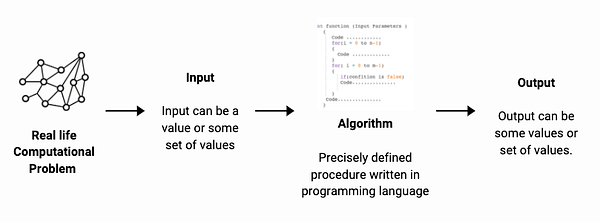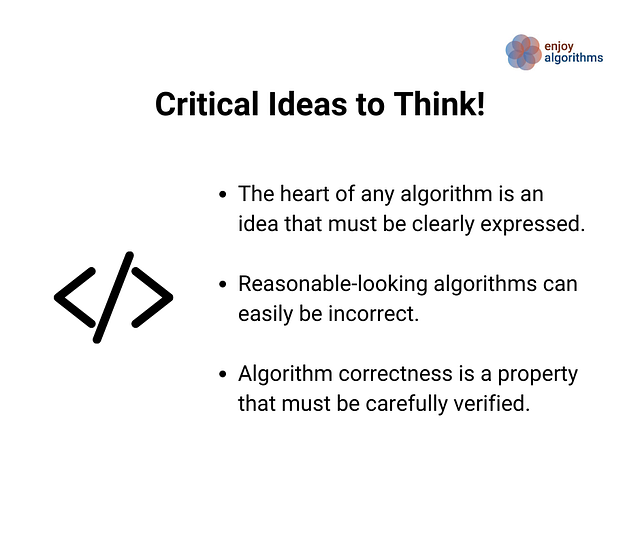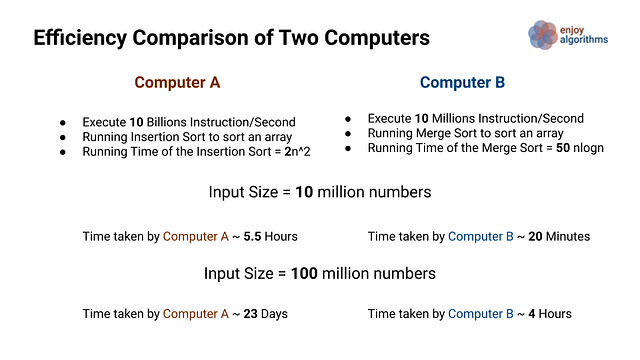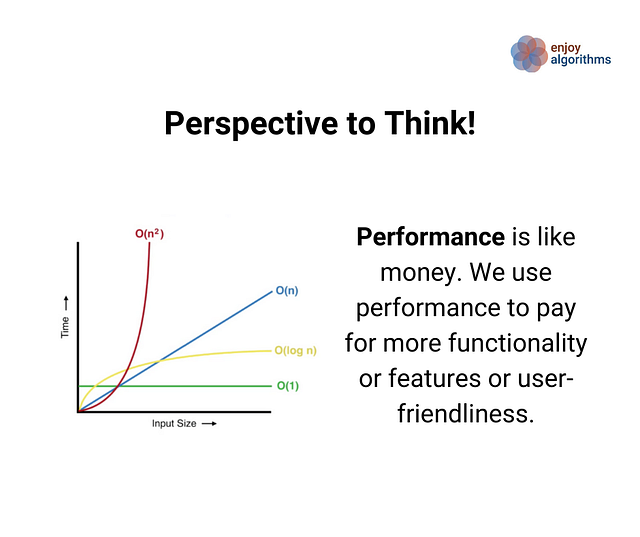# What is an Algorithm? Properties and Applications of Algorithms in real life

### Introduction to Algorithms

Before the invention of computers, there were algorithms. Now computers are everywhere, so algorithms are everywhere! Algorithms lie at the heart of computing. If we observe our surroundings, we can find several algorithms working to solve our daily life problems: Social media networks, GPS applications, Google search, e-commerce platforms, Netflix recommendation systems, etc. applications are powered by algorithms. Even it is also popular for coding interviews to get a high-paying job in the software industry. So learning algorithms is one of the critical career skills for programmers!

• Why did we use algorithms before the invention of computers?
• Why some of the ancient algorithms are still relevant?
• Algorithms are about computers or much more than that?

### Definition of an Algorithm

An algorithm is a well-defined step-by-step procedure to transform a given input inot the desired output to solve a computational problem. In other words, an algorithm is a tool for solving a well-specified computational problem. Note: The computational problem is a collection of questions that computers might be able to solve. For example, the problem of sorting : “Given a sequence of n integer, arrange it into increasing order” is a computational problem.### Example: Linear Search

Given an array A[] of n elements, write an algorithm to search a given element k in A[]. If the element k is present, return the index where it is present in the array; otherwise, return -1 or false.

Extracting all relevant details from the problem

• Input size or total elements in the input = n
• Input data structure : Array or sequential memory
• Input data type : Integer which can be both positive or negative.
• Input distribution or constraint :  There is no constraint given in the input. All integers are stored in random order.
• Input :  Array of integers and a value k
• Output :  If the value is present then return the index otherwise return -1

``````Input: X[] = [ 5, 8, - 3, - 4, 13, 9, - 17, 7, 12], k = 13
Output: 4
Explanation: Element 13 is present at index 4.

Input: X[] = [ 5, 8, - 3, - 4, 13, 9, - 17, 7, 12], k = 11
Output: -1
Explanation: Element 11 is not present in X[].
``````

Linear Search Idea

The value k can be present at any index in the array because we don’t know the input distribution. So a simple strategy would be :

• We run a loop to compare k with each element of X[].
• If k matches with an element X[i], we return the index i.
• If k doesn’t match with any of the elements, we return -1.

Linear Search Pseudocode

``````int linearSearch(int X[], int n, int k)
{
for(int i = 0; i < n; i = i + 1)
{
if(X[i] == k)
return i
}
return -1
}
``````

### Critical Ideas to Remember!

Always ask the following questions related to input for every coding problem:

• What is the size of the input? like 10 or 1000 or 10 billion!
• What is the data type of the input? It can be an integer, floating-point number, character, string, or boolean!
• How input values are stored? It can be stored in a data structure like an array, linked list, tree, graph, etc.
• Is there some information available for the distribution of the input? like values are stored in sorted order, input is allowed in a certain range, some permutation of the input is allowed only, etc.

### Properties of a Good Algorithm

A good algorithm must be correct, efficient, finite, and easy to implement.

• Clearly Specified Input: we need to know all relevant details like input data type, input size, data structure, input distribution, etc. Input can be a value or some set of values.
• Clearly Specified Output: we need to know all relevant details related to the output. Output can be a value or some set of values.
• Correctness: For every set of inputs, our algorithm must halt with the correct output. In other words, our algorithm must be correct. The incorrect algorithm might halt with incorrect answers or not halt at the correct output for some input values.
• Efficiency: Running time and memory are bounded resources and our algorithms must use them wisely. In simple words, our algorithm should be efficient in terms of time and memory!
• Finiteness: Our algorithm must terminate after a finite set of steps. We should always avoid infinite recursion or infinite loop condition.
• Simple and Elegant: An algorithm should be easy to understand and implement.### Why analysing efficiency is important?

Suppose computers were infinitely fast and computer memory was free. Would you have any reason to study algorithms? But the reality is that computers may be fast but not infinitely fast, and memory may be inexpensive but not free. So, running time and space are essential resources for defining the performance of the computer program. Think!

In computer science, these things are as crucial as an algorithm’s performance: Correctness of a code, Functionality, User Friendliness, Modularity, Scalability, Security, Maintainability, Programmers time, etc. But the critical question is : why do we analyze the performance of an algorithm? Here are a few important reasons:

• The performance draws a line between feasible and infeasible.
• It provides a clean standard to think about the program or system behavior.
• Performance is just like money where we use it to pay for more functionality or user-friendliness. For example, we code in Java or C++ for the OOPS features, even though Java or C++ is approx. 3 times slower than C. In other words, we are willing to pay the performance by a factor of 3 to get more functionalities.
• Speed is always fun and we love it!

Suppose we would like to run two different sorting algorithms on two different computers A and B, where computer B is 1000 times slower than computer A. For comparing the performances, we are running the slower sorting algorithm Insertion sort on faster computer A and running the faster sorting algorithm Merge sort on slower computer B.What difference do we observe? Still, computer B is taking much less time than computer A, if the input size is large. This gap will increase further if we increase the input size. Great! This would be one of the reasons for learning algorithms and their efficiency. Think!

### An Algorithm is a Technology!

There can be different solutions or algorithms for the same coding problem and these solutions may differ in terms of efficiency. These differences can be much more significant than differences due to hardware and software. So the system performance depends on choosing efficient algorithms as much as on choosing fast hardware. Even applications that do not require algorithm directly at the application level, relies heavily upon algorithms. For examples:

• Does the application require fast hardware? The hardware design used algorithms.
• Does the application depend upon the user interface? The design of the user interface relies on algorithms.
• Does the application rely on fast networking? Networking relies heavily on routing algorithms.

Overall, algorithms are at the core of almost all computer applications. Just as rapid innovations are being made in other computer technologies, they are also being made in algorithms!### Real-life Applications of Algorithms and Data Structures

Application of array

• Arranging a particular type of data in a sequential arrangement: storing contacts on our phone, storing speech signals in speech processing, etc.
• Implementing of Stack and Queue, Adjacency matrix representation of Graphs, Implementing hash tables and heaps.

• Singly-linked list: Maintaining a directory of names, Performing arithmetic operations on long integers, Manipulation of polynomials, Implementing of Stack and Queue, Adjacency list representation of Graphs, Hashing by chaining method, Dynamic memory allocation
• Doubly-linked list: Implementing LRU Cache, Implementing Fibonacci heap, Thread scheduler in operating systems, Representing deck of cards game, Implement undo and redo functionality in applications, Previous and next page in a web browser.
• Circular linked list: Processes scheduling in the operating system, Keep track of the turn in a multi-player game

Application of stack

• Storing browser history, UNDO/REDO options in a text editor
• Process scheduling, Static memory allocation
• Storing function calls in recursion
• In IDE or a compiler to know are missing braces
• Expression evaluation, Syntax Parsing

Application of queue

• Process scheduling in operating systems (CPU and IO scheduling)
• Interprocess communications
• Waiting list in Applications
• Customer care calls management

Application of hashing

• Accessing website using keywords in Search Engines
• Finding phone numbers on mobile devices, Employees Information System
• Spelling checkers in word processing software, Symbol table in a compiler
• Encrypting critical data(Cryptography)

Application of tree data structure

• Binary tree: Store hierarchical data, like folder structure, organization structure, File Systems in Linux, Document Object Model to represent documents with a logical tree
• Binary search tree: Used in applications where continuous insertion and deletion of data is happening, To implement map and set objects in languages' libraries.
• Trie data structure: Autocompletion in google search, spell-checking in word processors, Contact search on a phone book, swipe features in your mobile keypad, Network browser history of the URL's, Longest prefix matching used by routers in internet protocols, Predictive text technology (T9 dictionary in mobile phones)
• Heap data structure: Used in implementing efficient priority queues, scheduling processes in operating systems, Dynamic memory allocation, Order Statistics
• Advanced data structures: Compiler design for parsing of the syntax (Syntax Tree), Wireless networking and a memory allocation (Treap), Range query processing on a data set (Segment Tree or Binary Index Tree), finding the nearest neighbor to a particular point (K Dimensional Tree), To store data on the drive (B-tree), 3D computer graphics(BSP tree), Fast full-text search in word processors (Suffix tree)

Application of graph data structure

• Shortest path algorithm: Network routing protocols, Google maps, Shipment routing in e-commerce, Robot path planning
• Breadth-first search (BFS): Social network websites to find people with a given distance k from a person, Web crawling in a google search (BFS), Finding all neighbor nodes in the peer to peer network like BitTorrent (BFS), Network Broadcasting, Finding neighboring places via GPS navigation
• Depth-first search (DFS): Detecting cycle in a graph, Bipartiteness test in a graph, Finding strongly connected components, Solving maze puzzles, Topological sorting, Path-finding
• Topological sorting: Used in many different scenarios that involve scheduling several tasks with inter-dependencies. For example, 1) Generates a dependency graph of the dependent modules in IDE Build-Systems 2) Determining order to create complex database tables with interdependencies 3) Determining order to take courses and their pre-requisites to complete a degree.
• Other important applications: Assigning fastest pick-ups to Uber drivers (Hungarian algorithm), Facebook’s Friend Suggestion algorithm, Google Page Ranking algorithm where web pages are considered to be the vertices, Resource Allocation Graph (Operating System), Transaction graphs in cryptocurrency (Blockchain, which is a large graph), Artificial neural networks, Facebook Graph Search, Google Knowledge Graph, Product Recommendation Graphs (Amazon Recommendation System)

Application of dynamic programming

• Sequence Alignment, Document diffing Algorithms, Document Distance Algorithm (Edit Distance), Plagiarism Detection, a Typesetting system
• Duckworth Lewis Method in cricket, Flight control
• Speech recognition, Image processing, Machine learning algorithms
• Economics, Financial Trading, Bioinformatics, Operations research

Application of greedy algorithms

• Loss-less data compression of .png and .mp3 file-formats (Huffman Coding)
• Shortest Path Algorithms (Dijkstra Algorithms), Minimum spanning tree (Kruskal and Prim's algorithms), Approximation algorithms for NP-hard problems
• Solving activity selection and other optimization problems

Application of backtracking

• Solving famous puzzles like N-queens, crosswords, verbal arithmetic, Sudoku
• Solving various optimization and constraint satisfaction problem

Application of number theory

• Cryptography and Computer graphics
• Designing Hash functions and Random number generators
• Fast arithmetic operations

Other applications of algorithms and Data Structures

• Exact String Matching (KMP Algorithms)
• Image editing software like photoshop (Convex-hull algorithms)
• Fast search in a sorted dataset (Binary Search)
• Filter out stories that people have seen before (Quora uses a bloom filter for this)
• Breaking down signals into frequencies (Fast Fourier Transform)
• Block-sorting compression (Suffix Array)

### Coding Problems to Warm-up in Algorithms

You can find these problems on the Internet.

• Reverse an array, Find the transpose of a matrix
• Bubble sort, Selection sort, Insertion sort
• Convert Roman to Integer
• Find the GCD of two numbers
• Given a number n, check if it is prime or not
• Check if a given string is a palindrome
• Print a given matrix in spiral form
• Find the largest element in an array

### Content References

• Algorithms by CLRS
• The Algorithm Design Manual by Steven Skiena

Enjoy learning! Enjoy coding! Enjoy algorithms!